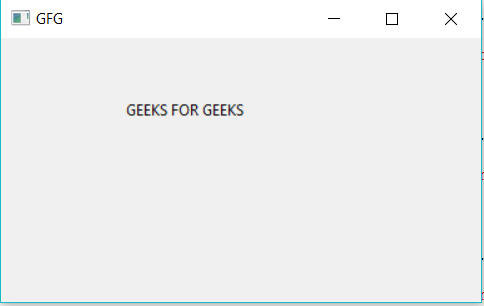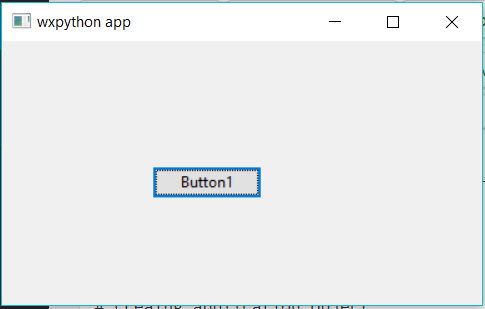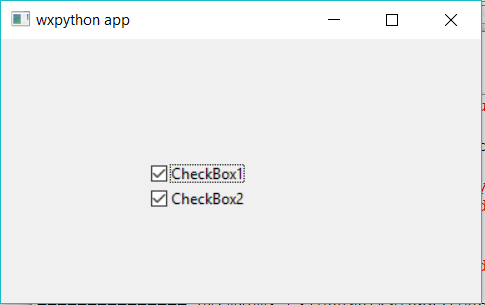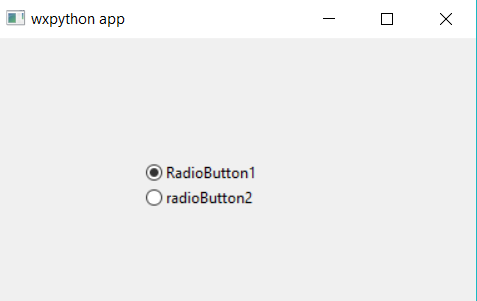Open In App

# Python | wxPython module Introduction

Python provides wxpython module which allows us to create high functional graphical user interface. It is an Open Source module, which means it is free for anyone to use and the source code is available for anyone to look and modify.
It is implemented as a set of extension modules that wrap the GUI components of wxWidgets library which is written in C++. It is cross platform GUI toolkit for python, Phoenix version Phoenix is the improved next-generation wxPython and it mainly focused on speed, maintain ability and extensibility.
Install using this command:

`pip install wxpython`

Creating GUI using wxpython:

1. First import wx module.
2. Create an object for application class.
3. Create an object for frame class and other controls are added to frame object so its layout is maintained using panel .
4. Then add a Static text object to show Hello World .
5. Show the frame window by using show method.
6. Run the app till the window is closed by using main event loop application object.

Example #1: A simple GUI application which says GEEKS FOR GEEKS using wxpython.

## Python3

 `# import wx module``import` `wx` `# creating application object``app1 ``=` `wx.App()` `# creating a frame``frame ``=` `wx.Frame(``None``, title ``=``"GFG"``)``pa ``=` `wx.Panel(frame)` `# Adding a text to the frame object``text1 ``=` `wx.StaticText(pa, label ``=``"GEEKS FOR GEEKS"``, pos ``=``(``100``, ``50``))` `# show it``frame.Show()` `# start the event loop``app1.Mainloop()`

Output:Example #2: Creating Buttons using wx module

## Python3

 `# Import wx module``import` `wx` `# creating application object``app1 ``=` `wx.App()` `# creating a frame``frame ``=` `wx.Frame(``None``, title ``=``"wxpython app"``)``pa ``=` `wx.Panel(frame)` `# Button creation``e ``=` `wx.Button(pa, ``-``1``, ``"Button1"``, pos ``=` `(``120``, ``100``))` `# show it``frame.Show()` `# start the event loop``app1.Mainloop()`

Output:Example #3: CheckBox using wxpython

## Python3

 `# importing wx module``import` `wx` `# creating application object``app1 ``=` `wx.App()` `# creating a frame``frame ``=` `wx.Frame(``None``, title ``=``"wxpython app"``)``pa ``=` `wx.Panel(frame)` `# Checkbox creation using wx module``e ``=` `wx.CheckBox(pa, ``-``1``, ``"CheckBox1"``, pos ``=` `(``120``, ``100``))``e ``=` `wx.CheckBox(pa, ``-``1``, ``"CheckBox2"``, pos ``=` `(``120``, ``120``))` `# show it``frame.Show()` `# start the event loop``app1.Mainloop()`

Output:`# importing wx module``import` `wx` `# creating application object``app1 ``=` `wx.App()` `# creating a frame``frame ``=` `wx.Frame(``None``, title ``=``"wxpython app"``)``pa ``=` `wx.Panel(frame)` `# RadioButton creation using wx module``e ``=` `wx.RadioButton(pa, ``-``1``, ``"RadioButton1"``, pos ``=` `(``120``, ``100``))``e ``=` `wx.RadioButton(pa, ``-``1``, ``"radioButton2"``, pos ``=` `(``120``, ``120``))` `# show it``frame.Show()` `# start the event loop``app1.Mainloop()`